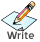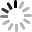잠시만 기다려 주세요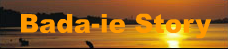HOME   |여기는?   |바다물때표   |여러가지 팁 (757)  |추천 및 재미 (15)  |자료실 (16)  |끄적거림 (414)  |시나리오 (757)  |드라마 대본 (248)  |
 2019년 03월 21일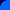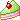모든 걸 다 잃고 나서야 비로소 나는, 사소한 것들의 소중함들을 깨달을 수 있게 되었다. - 바다아이

현재 접속자

인터넷 성경보기

소스 하이라이팅

도로명 주소검색

URL encode, decode

javascript 줄 맞추기

Donate 기부하기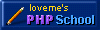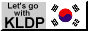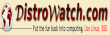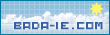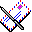Hits :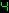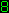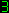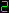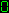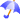golang

 golang - strconv.ParseInt strconv.Atoi strconv.FormatInt strconv.Itoa 정수 문자열 변환 이 름 : 바다아이   |   조회수 : 1452       ParseInt, strconv. A string contains digit characters like "123." We can convert this string to a number, an int, with the ParseInt method.
Other options. With Atoi we have a simpler way to call ParseInt. These methods return two values: the parsed integer itself, and an error code.
Example, ParseInt. Let us begin with this simple example. We import the strconv package at the top. We call ParseInt with three arguments—the first is the string.

Argument 2:This is the base of the string being parsed. Most numbers we use are base 10.

Argument 3:This is the bit size of the resulting int. 0 means int, while 6 (for example) means int16, with 16 bits.

Based on: Golang 1.7
Golang program that uses strconv.ParseInt  package main  import (     "fmt"     "strconv" )  func main() {     value := "123"     // Convert string to int.     number, _ := strconv.ParseInt(value, 10, 0)     // We now have an int.     fmt.Println(number)     if number == 123 { 	fmt.Println(true)     } }  Output  123 true

Atoi. This function bears the same name as the one from the C standard library. In Go it invokes ParseInt with a base of 10 and a bit size of 0.

Tip:The Atoi call here is simpler than ParseInt. This gives it an advantage in readability in programs.

Golang program that uses Atoi  package main  import (     "fmt"     "strconv" )  func main() {     value := "456"     // Use Atoi to parse string.     number, _ := strconv.Atoi(value)      fmt.Println(number)     fmt.Println(number + 1) }  Output  456 457

FormatInt. With this method, we convert an int to a string. This is the opposite conversion as ParseInt. With FormatInt, we must pass an int64 value—we cast in this example.
Golang program that uses strconv.FormatInt  package main  import (     "fmt"     "strconv" )  func main() {     value := 1055      // Convert int to string with FormatInt.     // ... First convert to int64.     result := strconv.FormatInt(int64(value), 10)     fmt.Println(result)     if result == "1055" { 	fmt.Println(true)     } }  Output  1055 true

Itoa. This method converts an int into a string. It calls FormatInt with a base of 10. It also accepts a simple int, not an int64, so less casting may be required.

Note:Unlike ParseInt and Atoi, FormatInt and Itoa do not return an error value. They succeed on all possible arguments.

Golang program that uses Itoa, converts int to string  package main  import (     "fmt"     "strconv" )  func main() {     value := 700      // Use Itoa on an int.     result := strconv.Itoa(value)     fmt.Println(result)      // The string has 3 characters.     fmt.Println(len(result)) }  Output  700 3

ParseInt benchmark. Often we need to convert a string into an integer. This benchmark compares ParseInt and Atoi. It converts "1234" into an integer with each method.

Result:No big performance differences were found. The methods performed about the same.

So:The best method to use is the one that has the correct behavior on all the data you want to convert.

Golang program that benchmarks ParseInt, Atoi  package main  import (     "fmt"     "strconv"     "time" )  func main() {     t0 := time.Now()     original := "1234";      // Version 1: use ParseInt.     for i := 0; i < 1000000; i++ { 	result, _ := strconv.ParseInt(original, 10, 0) 	if result != 1234 { 	    return 	}     }      t1 := time.Now()      // Version 2: use Atoi.     for i := 0; i < 1000000; i++ { 	result, _ := strconv.Atoi(original) 	if result != 1234 { 	    return 	}     }      t2 := time.Now()     // Results of benchmark.     fmt.Println(t1.Sub(t0))     fmt.Println(t2.Sub(t1)) }  Results  15.637ms    ParseInt 15.625ms    Atoi   출처 : https://www.dotnetperls.com/parseint-go
짧은 주소 : https://www.bada-ie.com/su/?631523067673 ParseInt, strconv. A string contains digit characters like "123." We can convert this string to a number, an int, with the ParseInt method. Other options. With Atoi we have a simpler way to call ParseInt. These methods return two values: the parsed integer itself, and an error code. Example, ParseInt. Let us begin with this simple example. We import the strconv package at the top. We call ParseInt with three arguments—the first is the string. Argument 2:This is the base of the string being parsed. Most numbers we use are base 10. Argument 3:This is the bit size of the resulting int. 0 means int, while 6 (for example) means int16, with 16 bits. Based on: Golang 1.7 ``` Golang program that uses strconv.ParseInt package main import ( "fmt" "strconv" ) func main() { value := "123" // Convert string to int. number, _ := strconv.ParseInt(value, 10, 0) // We now have an int. fmt.Println(number) if number == 123 { fmt.Println(true) } } Output 123 true``` Atoi. This function bears the same name as the one from the C standard library. In Go it invokes ParseInt with a base of 10 and a bit size of 0. Tip:The Atoi call here is simpler than ParseInt. This gives it an advantage in readability in programs. ```Golang program that uses Atoi package main import ( "fmt" "strconv" ) func main() { value := "456" // Use Atoi to parse string. number, _ := strconv.Atoi(value) fmt.Println(number) fmt.Println(number + 1) } Output 456 457``` FormatInt. With this method, we convert an int to a string. This is the opposite conversion as ParseInt. With FormatInt, we must pass an int64 value—we cast in this example. ```Golang program that uses strconv.FormatInt package main import ( "fmt" "strconv" ) func main() { value := 1055 // Convert int to string with FormatInt. // ... First convert to int64. result := strconv.FormatInt(int64(value), 10) fmt.Println(result) if result == "1055" { fmt.Println(true) } } Output 1055 true``` Itoa. This method converts an int into a string. It calls FormatInt with a base of 10. It also accepts a simple int, not an int64, so less casting may be required. Note:Unlike ParseInt and Atoi, FormatInt and Itoa do not return an error value. They succeed on all possible arguments. ```Golang program that uses Itoa, converts int to string package main import ( "fmt" "strconv" ) func main() { value := 700 // Use Itoa on an int. result := strconv.Itoa(value) fmt.Println(result) // The string has 3 characters. fmt.Println(len(result)) } Output 700 3``` ParseInt benchmark. Often we need to convert a string into an integer. This benchmark compares ParseInt and Atoi. It converts "1234" into an integer with each method. Result:No big performance differences were found. The methods performed about the same. So:The best method to use is the one that has the correct behavior on all the data you want to convert. ```Golang program that benchmarks ParseInt, Atoi package main import ( "fmt" "strconv" "time" ) func main() { t0 := time.Now() original := "1234"; // Version 1: use ParseInt. for i := 0; i < 1000000; i++ { result, _ := strconv.ParseInt(original, 10, 0) if result != 1234 { return } } t1 := time.Now() // Version 2: use Atoi. for i := 0; i < 1000000; i++ { result, _ := strconv.Atoi(original) if result != 1234 { return } } t2 := time.Now() // Results of benchmark. fmt.Println(t1.Sub(t0)) fmt.Println(t2.Sub(t1)) } Results 15.637ms ParseInt 15.625ms Atoi 출처 : https://www.dotnetperls.com/parseint-go ```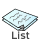|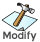|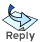제 목 이 름     1 page / 6 page 번 호 카테고리 제 목 이름 조회수 169 golang golang 파일 다운로드 예제... Force download file example 바다아이 40 168 golang golang 현재 하드웨어 및 사용현황 구하기, 하드웨어 모니터링, cpu, memory, disk 사용률 보기 바다아이 109 167 golang golang 원하는 형태로, 여러 종류로 크로스 컴파일 하기... GOOS, GOARCH 바다아이 159 166 golang golang 체크박스 checkbox 배열 폼 처리... 다중 체크박스 바다아이 218 165 golang golang 핸들함수, 라우터, http 웹서버 예제.... 바다아이 241 164 golang golang 에서 nil 을 할당할 수 있는 경우, nil 을 사용할 수 있는 타입, nil 을 사용... 바다아이 260 163 golang 2년 가까이 golang, go 언어를 사용해본 소감, 후기.... golang 찬양.... 추천 바다아이 433 162 golang golang 에서 postgresql, mysql, mariadb 다중쿼리 에러날 때... ` Grave (그레이브) 문제 될 때.. 쿼리문 에러.. 바다아이 338 161 golang golang 으로 postgresql 이나 mysql, mariadb 접속해서 데이터 가져올 때 scan 시 빈값, null 값이 오면 에러나는 문제.. 바다아이 379 160 golang golang 간편하게 문자 인코딩 변환, iconv 바다아이 430 159 golang golang quote escape, unquote 바다아이 440 158 golang golang 웹서버, http errorLog 에러로그 남기기, 저장하기, 기록하기... 바다아이 504 157 golang golang 인터페이스 interface 예제, 설명 바다아이 425 156 golang golang 구조체 struct 포인터 개념 정리.... 바다아이 494 155 golang golang map 안에 map 넣기, 만들기 출력하기 바다아이 469 154 golang golang map 값 조회하기.... 존재 유무 검사하기.... 바다아이 520 153 golang golang slice 배열 복사하기 copy 바다아이 440 152 golang golang goto 문 바다아이 495 151 golang golang slice 배열 sort 정렬, int, string, float64 바다아이 522 150 golang URL Parsing, url 파싱하기. parse 바다아이 529 149 golang golang 형변환, interface{} 요소를 형과 상관없이 문자열로 알아서 변환... 바다아이 495 148 golang golang interface to string, 인터페이스를 문자형, 문자열로 형 변환, map slice json 바다아이 465 147 golang golang How to convert JSON string to map and slice, json 컨버트 사용하기 바다아이 480 146 golang golang 파일 쓰기, file write, Writing Files 바다아이 619 145 golang golang Insert Line or String to File, 파일의 특정 행에, 라인에, 원하는 줄에 텍스트 삽입하기, 입력하기, 추가하기 바다아이 474 144 golang golang 문자열 치환하기 replace 바다아이 479 143 golang golang split explode 구분자를 통해 문자열 분할, 나누기, 자르기... 바다아이 521 142 golang golang email read parse, 이메일 읽기, 파싱..... 예제.... 바다아이 486 141 golang golang smtp email sendmail 이메일 보내기 바다아이 524 140 golang golang sort 정렬 예제, example, struct, 구조체 정렬 바다아이 553 123456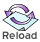| |